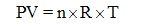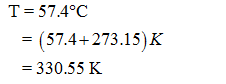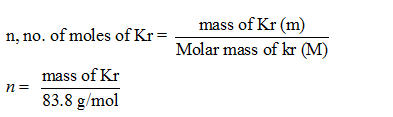# How many grams of Kr are in a 6.29 L cylinder at 57.4 ∘C and 8.15 atm?

Question
1 views

How many grams of Kr are in a 6.29 L cylinder at 57.4 ∘C and 8.15 atm?

check_circle

Step 1

We know the relation from gas law equation:Where,

P = pressure in atm;

V = volume in litres;

n = number of moles;

T = temperature in Kelvin;

R = gas constant = 0.0821 atm L/mol K.

Step 2

Given:Atomic weight of Kr = 83.8 g/mol

Pressure, P = 8.15 atm

Temperature, T = 330.55 K

Volume, V = 6.29 L

Gas constant, R = 0.0821 Latm/mol K

Step 3

To determine:

The mass of Kr...

### Want to see the full answer?

See Solution

#### Want to see this answer and more?

Solutions are written by subject experts who are available 24/7. Questions are typically answered within 1 hour.*

See Solution
*Response times may vary by subject and question.
Tagged in